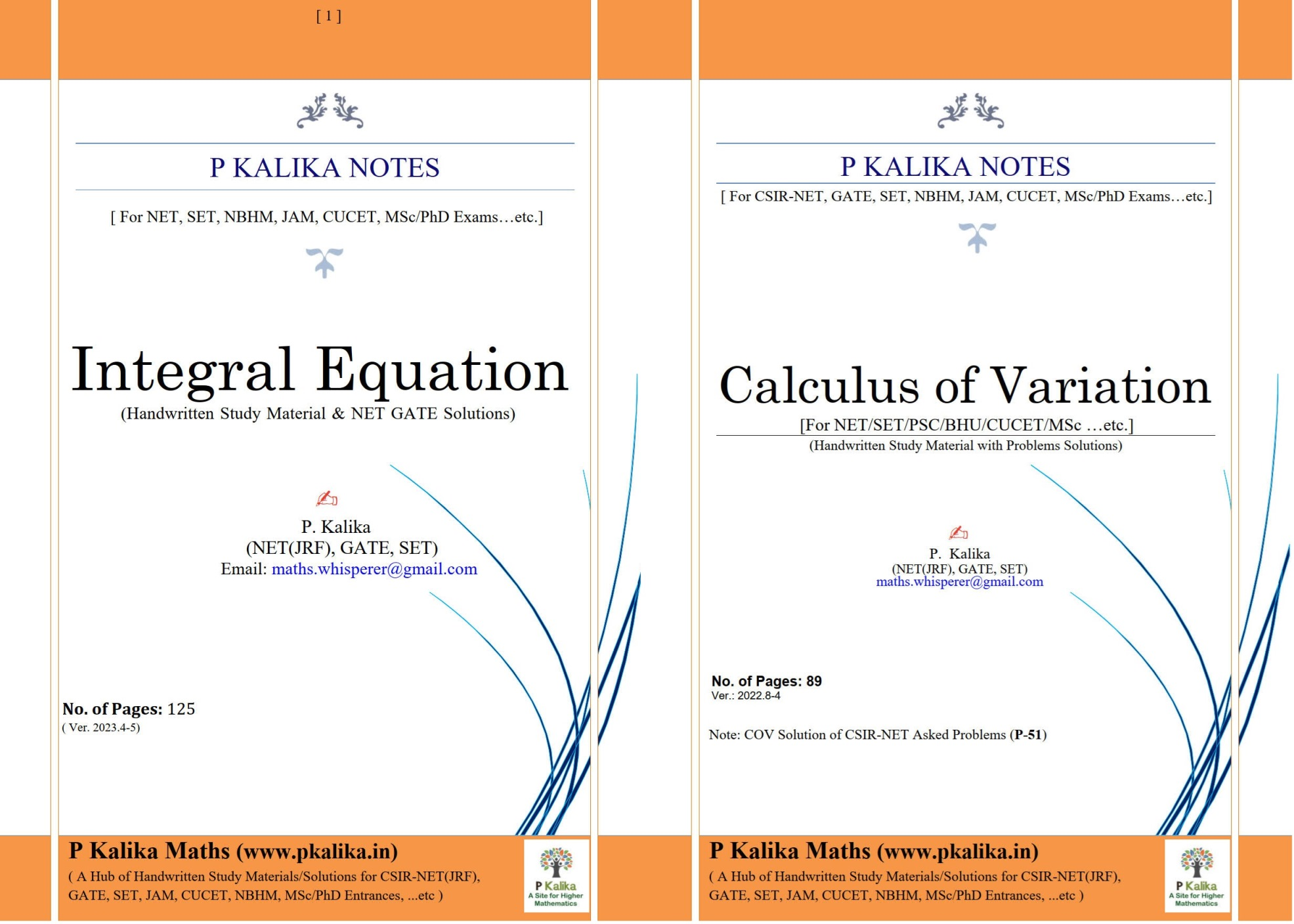P Kalika Maths Study Materials

# Integral Equation & Calculus of Variation for CSIR NET, SET, PSC, MSc.##### Integral Equation for CSIR NET, SET, PSC, MSc with Solved Problems
₹ 4549##### Calculus of Variation (COV) for CSIR NET, SET and PSC
₹ 37##### Integral Equation and COV CSIR NET Solutions
₹ 3943
Description

Integral Equation & Calculus of Variation handwritten study materials with CSIR-NET solutions for NET, SET, PSC, MSC, etc. exams.

In CSIR-NET, up to 12.50+12.50=25 marks questions are asked from Integral Equations and Calculus of Variation (COV).

Updated on: May 2023